## “ Stationarity and Non-Stationarity are terms you may have encountered previously in the case of Simple Harmonic Motion and other oscillation based theories. But what do these terms actually mean? A stationary time series has statistical properties or moments (e.g., mean and variance) that do not vary in time. Stationarity, then, is the status of a stationary time series. Conversely, Non-Stationarity is the status of a time series whose statistical properties are changing through time. ”

Background:

In time series analysis, we’d like to model the evolution of a time series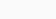from observations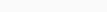. We particularly want to model moment functions of the time series. For instance, the mean function describes how the average value evolves over time, while the conditional mean function describes the same given past values. The autocovariance function describes the covariance between values at different points in time. We may also be interested in higher moment functions. When estimating parameters describing these, we’d like to be able to apply standard results from probability and statistics such as the law of large numbers and the central limit theorem.

If the moment functions are constant, then that greatly simplifies modeling. For instance, if the mean is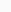instead of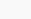then we estimate a constant rather than a function. This applies similarly to higher moments.

Definition of Stationarity:

A stochastic process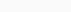is stationary if for any fixed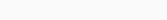does not change as a function of. In particular, moments and joint moments are constant. This can be described intuitively in two ways: 1) statistical properties do not change over time 2) sliding windows of the same size have the same distribution.

A simple example of a stationary process is a Gaussian white noise process, where each observation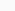is identical and independently distributed (iid)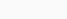. Let’s simulate Gaussian white noise which can be easily plotted on S2. Many stationary time series look somewhat similar to this when plotted:A simple example of a non-stationary process is a random walk. This is a sum of iid random variables with mean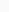. Example : Rademacher random variables (take values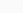each with probability) can be simulated using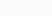steps and its plot looks like:It’s not super easy to see this from plots, but it can be shown mathematically that the variance of the time series increases over time, which violates stationarity.

Concept of Weak Sense Stationarity:

Often we are primarily interested in the first two moments of a time series: the mean and the autocovariance function. A process is weak sense or weakly stationary if: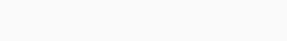– (1)

That is, if the mean does not depend on time and the auto-covariance between two elements only depends on the time between them and not the time of the first. An important motivation for this is Wold’s Theorem. This states that any weakly stationary process can be decomposed into two terms: a moving average and a deterministic process. Thus for a purely non-deterministic process we can approximate it with an ARMA process, the most popular time series model. Thus for a weakly stationary process we can use ARMA models. Intuitively, if a process is not weakly stationary, the parameters of the ARMA models will not be constant, and thus a constant model will not be valid.

Definition of Non-Stationarity:

Non-stationarity refers to any violation of the original assumption, but we’re particularly interested in the case where weak stationarity is violated. There are two standard ways of addressing it:

1. Assume that the non-stationarity component of the time series is deterministic, and model it explicitly and separately. This is the setting of a trend stationary model, where one assumes that the model is stationary other than the trend or mean function.

2. Transform the data so that it is stationary. An example is differencing which will be discussed later in the article.

Types of Non-Stationary Processes:

Non-stationary data, as a rule, are unpredictable and cannot be modeled or forecasted. The results obtained by using non-stationary time series may be spurious in that they may indicate a relationship between two variables where one does not exist. In order to receive consistent, reliable results, the non-stationary data needs to be transformed into stationary data. Before we get to the point of transformation for the non-stationary time series data, we should distinguish between the different types of non-stationary processes. This will provide us with a better understanding of the processes and allow us to apply the correct transformation.

Examples of non-stationary processes are random walk with or without a drift (a slow steady change) and deterministic trends (trends that are constant, positive, or negative, independent of time for the whole life of the series).• Pure Random Walk (Yt = Yt-1 + εt ) Random walk predicts that the value at time “t” will be equal to the last period value plus a stochastic (non-systematic) component that is a white noise, which means εt is independent and identically distributed with mean “0” and variance “σ².” Random walk can also be named a process integrated of some order, a process with a unit root or a process with a stochastic trend. It is a non-mean-reverting process that can move away from the mean either in a positive or negative direction. Another characteristic of a random walk is that the variance evolves over time and goes to infinity as time goes to infinity; therefore, a random walk cannot be predicted.

• Random Walk with Drift (Yt = α + Yt-1 + εt ) If the random walk model predicts that the value at time “t” will equal the last period’s value plus a constant, or drift (α), and a white noise term (εt), then the process is random walk with a drift. It also does not revert to a long-run mean and has variance dependent on time.

• Deterministic Trend (Yt = α + βt + εt ) Often a random walk with a drift is confused for a deterministic trend. Both include a drift and a white noise component, but the value at time “t” in the case of a random walk is regressed on the last period’s value (Yt-1), while in the case of a deterministic trend it is regressed on a time trend (βt). A non-stationary process with a deterministic trend has a mean that grows around a fixed trend, which is constant and independent of time.

• Random Walk with Drift and Deterministic Trend (Yt = α + Yt-1 + βt + εt ) Another example is a non-stationary process that combines a random walk with a drift component (α) and a deterministic trend (βt). It specifies the value at time “t” by the last period’s value, a drift, a trend, and a stochastic component.

Trend Stationarity:

A trend stationary stochastic process decomposes as: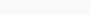– (2)

Here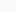is the deterministic mean function or trend and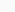is a stationary stochastic process. An important issue is that we need to specify a model for the mean function: generally we use a linear trend, possibly after a transformation (such as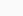).

Assuming that we know that trend stationarity holds, we do a three step process:

1. Fit a model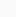to2. Subtractfrom, obtaining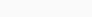3. Model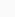(inference, forecasting, etc)

Let’s look at a synthetic example of a trend stationary process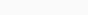where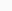iswhite noise. One can always try to plot it easily on S2:Corresponding to the plot above, the detrended series will look like:Trend and Difference Stationarity:

A random walk with or without a drift can be transformed to a stationary process by differencing (subtracting Yt-1 from Yt, taking the difference Yt – Yt-1) correspondingly to Yt – Yt-1 = εt or Yt – Yt-1 = α + εt and then the process becomes difference-stationary. The disadvantage of differencing is that the process loses one observation each time the difference is taken.A non-stationary process with a deterministic trend becomes stationary after removing the trend, or detrending. For example, Yt = α + βt + εt is transformed into a stationary process by subtracting the trend βt: Yt – βt = α + εt, as shown in the figure below. No observation is lost when detrending is used to transform a non-stationary process to a stationary one.In the case of a random walk with a drift and deterministic trend, detrending can remove the deterministic trend and the drift, but the variance will continue to go to infinity. As a result, differencing must also be applied to remove the stochastic trend.

Conclusion:

Using non-stationary time series data in some real-time models may produce unreliable and spurious results and leads to poor understanding and forecasting. The solution to the problem is to transform the time series data so that it becomes stationary. If the non-stationary process is a random walk with or without a drift, it is transformed to a stationary process by differencing. On the other hand, if the time series data analyzed exhibits a deterministic trend, the spurious results can be avoided by detrending.

Sometimes the non-stationary series may combine a stochastic and deterministic trend at the same time and to avoid obtaining misleading results both differencing and detrending should be applied, as differencing will remove the trend in the variance and detrending will remove the deterministic trend.